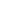Share

# Why are there 1024 bytes in a kilobyte?

Answer: Many people think that there are 1000 bytes in a kilobyte. After all, "kilo" means 1000. In most cases, this approximation is fine for determining how much space a file takes up or how much disk space you have.

But there are really 1024 bytes in a kilobyte. The reason for this is because computers are based on the binary system. That means hard drives and memory are measured in powers of 2. For example,

• 2^0 = 1
• 2^1 = 2
• 2^2 = 4
• 2^3 = 8
• 2^4 = 16
• 2^5 = 32
• 2^6 = 64
• 2^7 = 128
• 2^8 = 256
• 2^9 = 512
• 2^10 = 1024

Notice how 2^10 is 1024. Therefore, 2^10, or 1024 bytes compose one kilobyte. Furthermore, 1024 kilobytes compose one megabyte, and 1024 megabytes compose one gigabyte. For most practical purposes, you can estimate 1024 to 1000. But correcting someone who says there are 1000 bytes in a kilobyte is a great way to impress your friends.

#### Published: June 7, 2002 — by Per Christensson

Answer from the PC Help Center
https://pc.net/helpcenter/why_1024_bytes